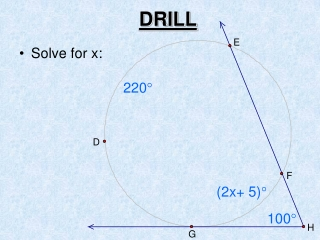DownloadDownload PresentationDRILL

# DRILL

Download Presentation## DRILL

- - - - - - - - - - - - - - - - - - - - - - - - - - - E N D - - - - - - - - - - - - - - - - - - - - - - - - - - -
##### Presentation Transcript

1. DRILL 220° • Solve for x: (2x+ 5)° 100°

2. 12.6 Segment Lengths in Circles Geometry Mr. Calise

3. Objectives/Assignment • Find the lengths of segments of chords. • Find the lengths of segments of tangents and secants.

4. Finding the Lengths of Chords • When two chords intersect in the interior of a circle, each chord is divided into two segments which are called segments of a chord. The following theorem gives a relationship between the lengths of the four segments that are formed.

5. If two chords intersect in the interior of a circle, then the product of the lengths of the segments of one chord is equal to the product of the lengths of the segments of the other chord. Theorem 12.14 EA • EB = EC • ED

6. Proving Theorem 12.14 • You can use similar triangles to prove Theorem 10.15. • Given: , are chords that intersect at E. • Prove: EA • EB = EC • ED

7. = Proving Theorem 12.14 Paragraph proof: Draw and . Because C and B intercept the same arc, C  B. Likewise, A  D. By the AA Similarity Postulate, ∆AEC  ∆DEB. So the lengths of corresponding sides are proportional. Lengths of sides are proportional. EA • EB = EC • ED Cross Product Property

8. Chords ST and PQ intersect inside the circle. Find the value of x. RQ• RP =RS •RT Use Theorem 10.15 9• x = 3 • 6 Substitute values. Simplify. 9x = 18 Divide each side by 9. x = 2 Ex. 1: Finding Segment Lengths

9. 12 2x 10 15

10. In the figure shown, PS is called a tangent segment because it is tangent to the circle at an end point. Similarly, PR is a secant segment and PQ is the external segment of PR. Segments of Tangents and Secants

11. If a secant segment and a tangent segment share an endpoint outside a circle, then the product of the length of the secant segment and the length of its external segment equal the square of the length of the tangent segment. Theorem 12.16 (EA)2 = EC • ED

12. 10 6 x

13. 8 12

14. Finding Segment Lengths (BA)2 = BC • BD Use Theorem 10.17 (5)2 =x • (x + 4) Substitute values. Simplify. 25 = x2 + 4x Write in standard form. 0 = x2 + 4x - 25 Use Quadratic Formula. x = x = Simplify. Use the positive solution because lengths cannot be negative. So, x = -2 +  3.39.

15. If two secant segments share the same endpoint outside a circle, then the product of the length of one secant segment and the length of its external segment equals the product of the length of the other secant segment and the length of its external segment. Theorem 12.15 EA• EB = EC • ED

16. Find the value of x. Finding Segment Lengths RP • RQ = RS • RT Use Theorem 10.16 9•(11 + 9)=10•(x + 10) Substitute values. 180 = 10x + 100 Simplify. 80 = 10x Subtract 100 from each side. 8 = x Divide each side by 10.

17. 10 6 x 15

18. Aquarium Tank. You are standing at point C, about 8 feet from a circular aquarium tank. The distance from you to a point of tangency is about 20 feet. Estimate the radius of the tank. Ex. 3: Estimating the radius of a circle

19. Solution (CB)2 = CE • CD Use Theorem 10.17 (20)28 • (2r + 8) Substitute values. Simplify. 400 16r + 64 Subtract 64 from each side. 336  16r Divide each side by 16. 21  r So, the radius of the tank is about 21 feet.

20. Congratulationson Finishing the Geometry Curriculum • Chapter 12 Test on Wednesday! • REVIEW Days June 3rd, 4th, 7th, 8th, 9th, 10th, 11th,14th (PD 3 Only), 15th (PD 3 Only) • FINAL EXAM • PD 2: Tuesday June 15th (7:55 – 9:30) • PD 3: Tuesday June 15th (12:30 – 2:05)